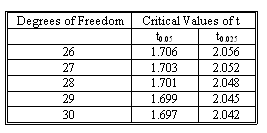### CFA Practice Question

There are 208 practice questions for this study session.

### CFA Practice Question

In a multiple regression model based on 30 observations and 3 independent variables, you have the estimate of b1 equal to 1.35. The standard error of this estimate is .13. Find the 95% confidence interval for the parameter b1.A. [1.083, 1.617].
B. [1.084, 1.616].
C. [1.128, 1.572].
Explanation: The degrees of freedom equals 26. Therefore, the critical value of t for a 95% confidence interval will be 2.056 (the 5% probability will be split between the tails). Thus, the confidence interval will be [1.35 - 0.13 x 2.056, 1.35 + 0.13 x 2.056] = [1.083, 1.617].